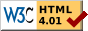#Boost C++ Libraries

...one of the most highly regarded and expertly designed C++ library projects in the world.

This is the documentation for a snapshot of the develop branch, built from commit f7b3e24375.

# Numbers Requirements

What we call "number" is the base type of the `interval` class. The interval library expect a lot of properties from this base type in order to respect the inclusion property. All these properties are already detailed in the other sections of this documentation; but we will try to summarize them here.

### Ordering

The numbers need to be supplied with an ordering. This ordering expresses itself by the operators `< <= => > == !=`. It must be a total order (reflexivity, antisymmetry, transitivity, and each pair of numbers is ordered). So `complex<T>` will not be a good candidate for the base type; if you need the inclusion property of interval property, you should use ```complex< interval<T> >``` in place of `interval< complex<T> >` (but unfortunately, `complex` only provides specialization).

Please note that invalid numbers are not concerned by the order; it can even be conceptually better if a comparison with these invalid numbers is always `false` (except for `!=`). If your checking policy uses `interval_lib::checking_base` and your base type contains invalid numbers, then this property is needed: `nan!=nan` (here `nan` is an invalid number). If this property is not present, then you should not use `checking_base` directly.

Interval arithmetic involves a lot of comparison to zero. By default, they are done by comparing the numbers to `static_cast<T>(0)`. However, if the format of the numbers allows some faster comparisons when dealing with zero, the template functions in the `interval_lib::user` namespace can be specialized:

```namespace user {
template<class T> inline bool is_zero(T const &v) { return v == static_cast<T>(0); }
template<class T> inline bool is_neg (T const &v) { return v <  static_cast<T>(0); }
template<class T> inline bool is_pos (T const &v) { return v >  static_cast<T>(0); }
}
```

### Numeric limits

Another remark about the checking policy. It normally is powerful enough to handle the exceptional behavior that the basic type could induce; in particular infinite and invalid numbers (thanks to the four functions `pos_inf`, `neg_inf`, `nan` and `is_nan`). However, if you use `interval_lib::checking_base` (and the default checking policy uses it), your base type should have a correctly specialized `std::numeric_limits<T>`. In particular, the values `has_infinity` and `has_quiet_NaN`, and the functions `infinity` and `quiet_NaN` should be accordingly defined.

So, to summarize, if you do not rely on the default policy and do not use `interval_lib::checking_base`, it is not necessary to have a specialization of the numeric limits for your base type.

### Mathematical properties

Ensuring the numbers are correctly ordered is not enough. The basic operators should also respect some properties depending on the order. Here they are:

• 0 ≤ x ⇒ -x ≤ 0
• xy ⇒ -y ≤ -x
• xyx+zy+z
• xy and z ≥ 0 ⇒ x×zy×z
• 0 < xy ⇒ 0 < 1/y ≤ 1/x

The previous properties are also used (and enough) for `abs`, `square` and `pow`. For all the transcendental functions (including `sqrt`), other properties are needed. These functions should have the same properties than the corresponding real functions. For example, the expected properties for `cos` are:

• `cos` is defined for all the valid numbers;
• it is 2π-periodic;
• `cos`(2π-x) is equal to `cos`(x);
• `cos` is a decreasing function on [0,2π].

### Rounding

If you work with a base type and no inexact result is ever computed, you can skip the rest of this paragraph. You can also skip it if you are not interested in the inclusion property (if approximate results are enough). If you are still reading, it is probably because you want to know the basic properties the rounding policy should validate.

Whichever operation or function you consider, the following property should be respected: `f_down(x,y) <= f(x,y) <= f_up(x,y)`. Here, `f` denotes the infinitely precise function computed and `f_down` and `f_up` are functions which return possibly inexact values but of the correct type (the base type). If possible, they should try to return the nearest representable value, but it is not always easy.

### Constants

In order for the trigonometric functions to correctly work, the library need to know the value of the π constant (and also π/2 and 2π). Since these constants may not be representable in the base type, the library does not have to know the exact value: a lower bound and an upper bound are enough. If these values are not provided by the user, the default values will be used: they are integer values (so π is bounded by 3 and 4).

### Operators and conversions

As explained at the beginning, the comparison operators should be defined for the base type. The rounding policy defines a lot of functions used by the interval library. So the arithmetic operators do not need to be defined for the base type (unless required by one of the predefined classes). However, there is an exception: the unary minus need to be defined. Moreover, this operator should only provide exact results; it is the reason why the rounding policy does not provide some negation functions.

The conversion from `int` to the base type needs to be defined (only a few values need to be available: -1, 0, 1, 2). The conversion the other way around is provided by the rounding policy (`int_down` and `int_up` members); and no other conversion is strictly needed. However, it may be valuable to provide as much conversions as possible in the rounding policy (`conv_down` and `conv_up` members) in order to benefit from interval conversions.Revised 2006-12-24